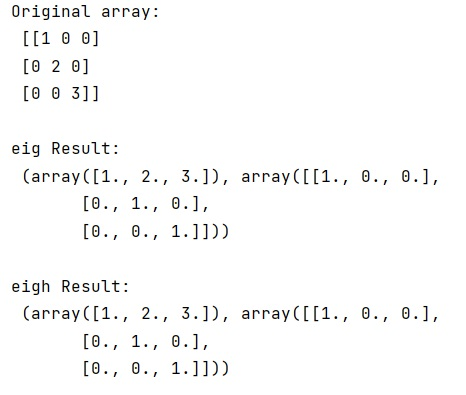# Difference between linalg.eig() and linalg.eigh() in Python NumPy

In this tutorial, we will learn what's the difference between linalg.eig() and linalg.eigh() functions in Python NumPy? By Pranit Sharma Last updated : April 08, 2023

The numpy.linalg is used to call linear algebra function to carry out linear algebra operation.

## Difference between linalg.eig() and linalg.eigh()

The eigh() guarantees us that the eigenvalues are sorted and uses a faster algorithm that takes advantage of the fact that the matrix is symmetric. If we know that our matrix is symmetric, we should use this function.

Although, eigh() does not check if our matrix is indeed symmetric, it by default just takes the lower triangular part of the matrix and assumes that the upper triangular part is defined by the symmetry of the matrix.

On the other hand, the eig() function works for general matrices and therefore uses a slower algorithm, we can check that for example with IPython's magic command %timeit. If we test with larger matrices, we will also see that in general the eigenvalues are not sorted here.

Let us understand with the help of an example,

## Python code to demonstrate the difference between linalg.eig() and linalg.eigh() functions

```# Import numpy
import numpy as np

# Creating an array
arr = np.diag((1, 2, 3))

# Display array
print("Original array:\n",arr,"\n")

# linalg eig
res = np.linalg.eig(arr)

# Display result
print("eig Result:\n",res,"\n")

# linalg eigh
res = np.linalg.eigh(arr)

# Display result
print("eigh Result:\n",res)
```

### Output